# The mxnorm package for removing slide-to-slide variation in multiplexed imaging data

This vignette describes the mxnorm R package, and provides example analyses & detailed methodological background for using normalization methods in multiplexed imaging data. The package largely provides two key services: (1) a collection of normalization methods and analysis metrics to implement and compare normalization in multiplexed imaging data, and (2) a foundation for storing multiplexed imaging data in R using S3. Background for these methods and the multiplexed imaging field can be found in the author’s previously published work (Harris et al. 2022).

## Basic example

This vignette using the following packages:

library(mxnorm)
library(dplyr)
library(reticulate)

We also use the Python module scikit-image.filters later on in this vignette – the mxnorm package has been configured to try and avoid any conflicts with installing this module.

if(reticulate::py_module_available("skimage")){
knitr::knit_engines$set(python = reticulate::eng_python) skimage_available = TRUE } else{ ## set boolean for CRAN builds skimage_available = FALSE ## install if running locally #py_install("scikit-image") } If you are prompted to install Miniconda, please do so. Please also see the reticulate package documentation for more information if you run into issues. ### Loading in data In general, we expect multiplexed imaging data in a data.frame format that includes columns for slide & image identifiers, separate columns for marker intensity values, and some set of metadata columns like tissue identifiers, phenotypic traits, medical conditions, etc. Alongside the mxnorm package itself, we introduce the mx_sample dataset that demonstrates the expected structure of multiplexed imaging data, and provides simulated marker intensity values that demonstrate strong slide effects. This structure is as follows: head(mx_sample, 3) #> slide_id image_id marker1_vals marker2_vals marker3_vals metadata1_vals #> 1 slide1 image1 15 17 28 yes #> 2 slide1 image1 11 22 31 no #> 3 slide1 image1 12 16 22 yes This dataset consists of 3 markers across 4 slides (with 750 “cells” on each slide) and 1 metadata column, and was specifically created to demonstrate the effect of normalization methods in multiplexed imaging data. To ensure a streamlined framework for the analysis of this type of data, we have created an S3 object mx_dataset to store the data and continue building upon as we normalize the data and analyze our results. ### Creating the S3 object with mx_dataset() Let’s load the mx_sample dataset into the S3 object we’ll use for our analyses, the mx_dataset object. Here we specify: • data: the input dataset in a data.frame format • slide_id and image_id: the identifiers of interest in our dataset • marker_cols: the set of marker columns in our input data that we want to include • metadata_cols: metadata columns in our input data that we want to include mx_data = mx_dataset(data=mx_sample, slide_id="slide_id", image_id="image_id", marker_cols=c("marker1_vals","marker2_vals","marker3_vals"), metadata_cols=c("metadata1_vals")) And now the mx_dataset S3 object becomes the foundation for each of the methods and analyses we have implemented in mxnorm. More information about the functionality of this package can be found in the Package overview. ### Normalization with mx_normalize() Now that we’ve created the object, we can use the mx_normalize() function to normalize the imaging data. Here we specify: • mx_data: the mx_dataset object with the data we want to normalize • transform: the transformation method we want to perform, which in this case is mean_divide. • method: the normalization method we want to implement, which in this case is None. • method_override: an optional parameter to provide a user-defined normalization method (see the details below for an example) • method_override_name: an optional parameter to re-name the method attribute when specifying user-defined normalization Note that there are multiple normalization approaches implemented into the mxnorm package (including user-defined normalization), which are discussed below in the Package overview and Methodology background sections. mx_data = mx_normalize(mx_data = mx_data, transform = "mean_divide", method="None", method_override=NULL, method_override_name=NULL) The mx_dataset object now has normalized data in the following form, with additional transform and method attributes: head(mx_data$norm_data,3)
#>   slide_id image_id marker1_vals marker2_vals marker3_vals metadata1_vals
#> 1   slide1   image1    0.6293173    0.4091531    0.5264357            yes
#> 2   slide1   image1    0.3063198    0.6621725    0.6367893             no
#> 3   slide1   image1    0.3870692    0.3585492    0.3057285            yes

### Otsu discordance scores with run_otsu_discordance()

Now that we’ve normalized the multiplexed imaging data, we can start to analyze the results and understand the performance of our normalization. Using the above normalized data, we can run an Otsu discordance score analysis to determine how well our normalization method performs. Note that below we set a boolean in case the machine building the vignette does not have the Python module scikit-image installed, and use the median as the threshold instead.

Broadly, this method calculates the distance of slide-level Otsu thresholds from the “global” Otsu threshold for a given marker channel to quantify the slide-to-slide alignment of values via a summary metric. In this analysis, we look for lower discordance scores to distinguish better performing normalization methods, which indicates better agreement between slides for a given marker.

To run this analysis we specify:

• mx_data: the mx_dataset object with the data we want to analyze
• table: the set of data we want to analyze using Otsu discordance, either raw, normalized, or both
• threshold_override: an optional parameter to provide a user-defined thresholding method (see the details below for example)
• plot_out: an optional parameter to output plots when running Otsu discordance
if(skimage_available){
thold_override = NULL
} else{
thold_override = function(thold_data){quantile(thold_data, 0.5)}
}

mx_data = run_otsu_discordance(mx_data,
table="both",
threshold_override = thold_override,
plot_out = FALSE)

This method adds an otsu_data table to the mx_dataset object that contains the results of the discordance analysis, with an additional attribute threshold to denote the type of thresholding algorithm used and the otsu_table to denote which tables in our object we ran the analysis on:

head(mx_dataotsu_data, 3) #> slide_id marker table slide_threshold marker_threshold #> 1 slide1 marker1_vals raw 12.01758 54.89844 #> 2 slide2 marker1_vals raw 20.01367 54.89844 #> 3 slide3 marker1_vals raw 87.05664 54.89844 #> discordance_score #> 1 0.4506667 #> 2 0.4306667 #> 3 0.2573333 We see in the above table that for each slide and marker pair, we generate a discordance_score that summarizes the distance between the slide_threshold and marker_threshold. Since we have completed this analysis, we can also begin to visualize some of the results. First, we plot the densities of each marker before and after normalization, along with the associated Otsu thresholds visible as a ticks in the rug plot for each density curve: plot_mx_density(mx_data)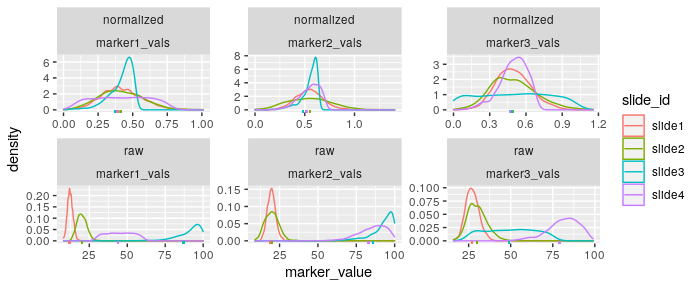In the above plot we observe that not only are the density curves for each marker in the analysis far better aligned after normalization, we also see that the Otsu thresholds (ticks on the x-axis) have moved far closer than in the raw data. In general, also note that all plots generated using mxnorm are ggplot2 plots and can be adjusted and adapted as needed given the ggplot2 framework. We can also visualize the results of the threshold discordance analysis stratified by slide and marker, with slide means indicated by the white diamonds: plot_mx_discordance(mx_data)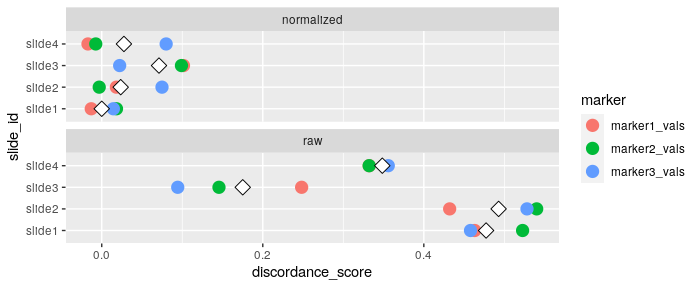Note that for each slide and marker pair in the dataset (denoted as colored points in the above plot), we see a reduction in threshold discordance in the normalized data compared to the raw data. Further, we also see dramatic improvements in the mean threshold discordance denoted by the white diamonds for the normalized data. ### UMAP dimension reduction with run_reduce_umap() We can also use the UMAP algorithm to reduce the dimensions of our markers in the dataset as follows, using the metadata_col parameter for later (e.g., this metadata is similar to tissue type, medical condition, subject group, etc. in practice). The UMAP algorithm is a random algorithm, so we use set.seed() below to ensure results are reproducible – further information on this algorithm can be found in the Methodology background. Here we specify: • mx_data: the mx_dataset object with the data we want to analyze • table: the set of data we want to analyze using UMAP dimension reduction, either raw, normalized, or both • marker_list: the markers in the mx_dataset object we want to use for dimension reduction • downsample_pct: UMAP embedding can be computationally expensive for big datasets, so we present a downsample percentage to reduce the input data size • metadata_col: any metadata in mx_dataset to store for plotting later (see below for plotting using metadata1_vals) set.seed(1234) mx_data = run_reduce_umap(mx_data, table="both", marker_list = c("marker1_vals","marker2_vals","marker3_vals"), downsample_pct = 0.5, metadata_cols = c("metadata1_vals")) This adds UMAP dimensions to our mx_dataset object in the following form (note the inclusion of slide_id as an identifier, which we’ll use later) and the umap_table attribute to denote which tables in our object we ran the analysis on. We can observe this data, and note the inclusion of UMAP coordinates: head(mx_dataumap_data, 3)
#>      marker1_vals marker2_vals marker3_vals metadata1_vals slide_id table
#> 1004           22           22           30             no   slide2   raw
#> 623            12           19           28            yes   slide1   raw
#> 2953           60           89           91            yes   slide4   raw
#>             U1         U2
#> 1004  -3.63464 -0.9834719
#> 623  -10.35462 -2.0627188
#> 2953  10.10994 -4.7028897

We can further visualize the results of the UMAP dimension reduction as follows using the metadata column we specified above:

plot_mx_umap(mx_data,
metadata_col = "metadata1_vals")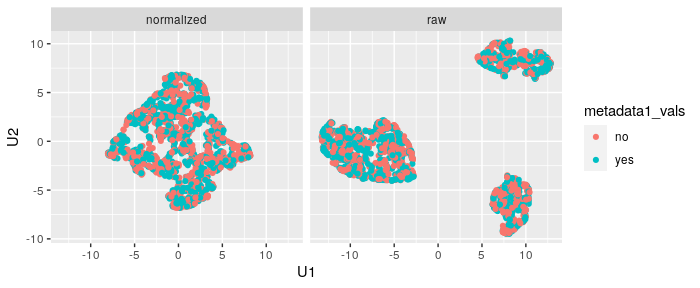Note that since the sample data is simulated, we don’t see separation of the groups like we would expect with biological samples that have some underlying correlation. What we can observe, however, is the clear separation of slides in the raw data and subsequent mixing of these slides in the normalized data:

plot_mx_umap(mx_data,
metadata_col = "slide_id")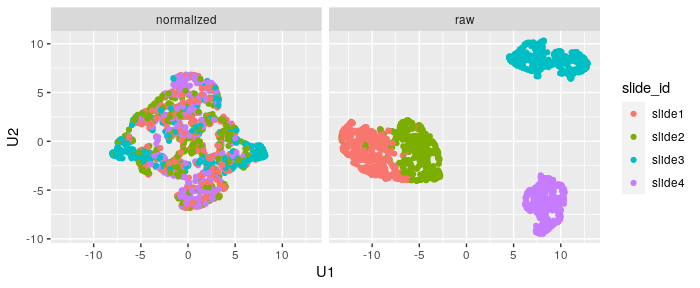### Variance components analysis with run_var_proportions()

We can also leverage lmer() from the lme4 package to perform random effects modeling on the data to determine how much variance is present at the slide level. The default model specified is as follows for each marker in the mx_dataset object (e.g. a random intercept model where the intercept is slide_id for each marker), with any specifications of metadata_cols in the run_var_proportions() call adding fixed effects into the model below:

$\text{marker} \sim \text{metadata_cols} + (1 | \text{slide_id})$ Note that the model we fit below sets the metadata_cols to NULL, implying the following basic random intercepts model:

$\text{marker} \sim (1 | \text{slide_id})$ In general, for an effective normalization algorithm we seek a method that reduces the proportion of variance at the slide level after normalization. Here we specify the following to run this analysis:

• mx_data: the mx_dataset object with the data we want to analyze
• table: the set of data we want to analyze using random effects, either raw, normalized, or both
• metadata_cols: any metadata in mx_dataset to add as fixed effects covariates,
• formula_override: an optional parameter to provide a user-defined random effects formula (see the details below for example)
• save_models: an optional parameter to save the lme4 models in the mx_dataset object
mx_data = run_var_proportions(mx_data,
table="both",
formula_override = NULL,
save_models = FALSE
)

Note that because the default model looks specifically at slide-level random effects, there are often errors of the form:

boundary (singular) fit: see ?isSingular

This can be remediated with proper specification of a robust random effects model using the formula_override option. After running the analysis, we see the addition of variance proportions to our mx_dataset object in the following form:

head(mx_data$var_data, 4) #> proportions level marker table #> 1: 0.97044933 slide marker1_vals raw #> 2: 0.02955067 residual marker1_vals raw #> 3: 0.97345576 slide marker2_vals raw #> 4: 0.02654424 residual marker2_vals raw These values summarize the proportion of variance explained by the random effect for slide, and any residual variance in the model. To understand how normalization impacts these values, we can further visualize these proportions as follows: plot_mx_proportions(mx_data)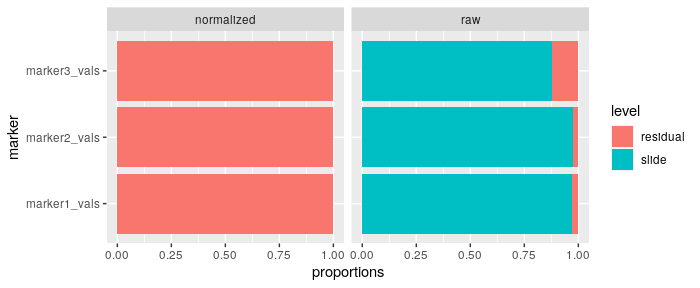Here we see that most of the variance in these models is due to slide-level effects in the raw data, but after normalization, nearly all of the variance in these random effects models due to slide-level effects is removed. This points to a normalization method that is performing well and removing the slide-to-slide variation in this type of data. ### Basic example overview We can use now also use the generic summary() function that the mxnorm package extends to mx_dataset objects to capture some values about this S3 object, including Anderson-Darling test statistics, thresholding summaries, consistency scores for the UMAP clustering results, and summaries of the variance proportions analysis. More information about some of these statistics can be found in the Methodology background. summary(mx_data) #> Call: #> mx_dataset object with 4 slide(s), 3 marker column(s), and 1 metadata column(s) #> #> Normalization: #> Data normalized with transformation=mean_divide and method=None #> #> Anderson-Darling tests: #> table mean_test_statistic mean_std_test_statistic mean_p_value #> normalized 36.845 25.852 0 #> raw 32.490 22.525 0 #> #> Threshold discordance scores: #> table mean_discordance sd_discordance #> normalized 0.031 0.042 #> raw 0.373 0.141 #> #> Clustering consistency (UMAP): #> table adj_rand_index cohens_kappa #> normalized 0.068 0.070 #> raw 0.881 -0.293 #> #> Variance proportions (slide-level): #> table mean sd #> normalized 0.00 0.000 #> raw 0.94 0.055 Because this is a toy example generated to demonstrate these effects, we see significant reduction in the normalized results – practically all of the metrics & statistics point to a reduction in slide-to-slide variation while preserving biological signal in the data. In general, one can reproduce an analysis using the following steps: ## create S3 object & normalize mx_data = mx_dataset(data, slide_id, image_id, marker_cols, metadata_cols) mx_data = mx_normalize(mx_data, transform, method) ## run analyses mx_data = run_otsu_discordance(mx_data, table) mx_data = run_reduce_umap(mx_data, table, marker_list, downsample_pct, metadata_cols) mx_data = run_var_proportions(mx_data,table) ## results and plots summary(mx_data) plot_mx_density(mx_data) plot_mx_discordance(mx_data) plot_mx_umap(mx_data) plot_mx_proportions(mx_data) ## Package overview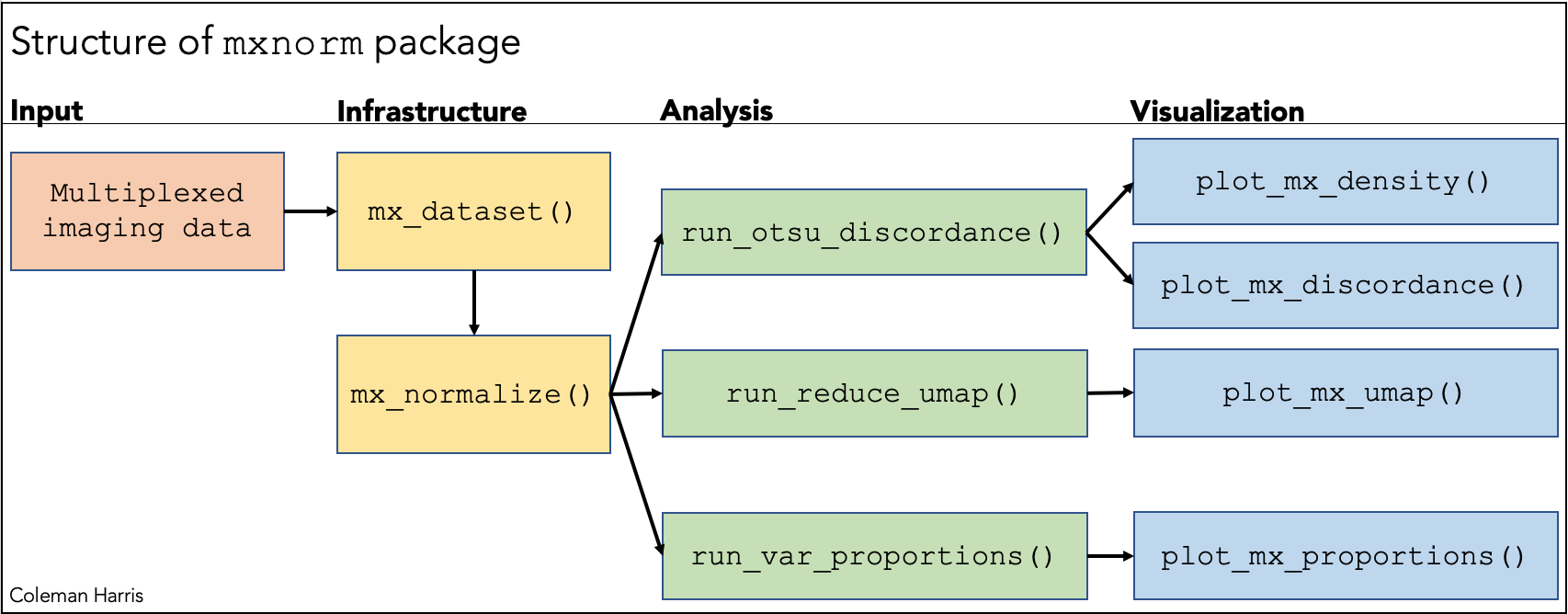Basic structure of the mxnorm package and associated functions ### Infrastructure functions (mx_) As noted above, the foundation of the mxnorm analyses is the mx_dataset S3 object. Here we introduce two important functions for handling multiplexed imaging data. First, to create the mx_dataset S3 object, we must call the mx_dataset() function, and to run any normalization we must run mx_normalize(). These are both required before any further analyses can be run. The constructor for the S3 object, mx_dataset() requires the following parameters to create & store the object: • data: The multiplexed data to normalize, assumed to be a data.frame with cell-level data. • slide_id: String slide identifier of input data • image_id: String image identifier of input data • marker_cols: Vector of column name(s) in the input data corresponding to marker values. • metadata_cols: Vector of other column name(s) in the input data to include as metadata. This parameter is optional, and defaults to NULL. After we create this S3 object, we can then run the normalization, analysis, and visualize our results using the other exposed functions in mxnorm. First, we must run the normalization of the data itself via the mx_normalize() method. Here, we leverage the S3 structure of the mx_dataset object to build upon and add attributes to keep our analysis in one consistent object. As described in our paper (Harris et al. 2022), we implement two discrete components of normalization of the multiplexed imaging data – transformation method and normalization method. Here, transformations change the “scale” of the data, of which the following are options for the transform parameter: c("None", "log10", "mean_divide","log10_mean_divide"). Here the log10 transformation can be represented mathematically as $$\log_{10}(y + 1)$$ for some intensity values $$y$$, the mean_divide method as $$\frac{y}{\mu}$$ for a slide mean of a given marker defined as $$\mu$$, and the log10_mean_divide method as $$\log_{10}\left(\frac{y}{\mu} + \frac{1}{2}\right)$$. Normalization methods are algorithms designed to normalize the data itself via some further set of assumptions, of which the following are options for the method parameter: c("None", "ComBat","Registration"). Further information about these methods and their implementations are discussed below in the Methodology background section. Note also the method_override parameter in the mx_normalize() function, that allows users to supply user-defined function to perform their own normalization methods, which is show below in the Use cases section. ### Analysis functions (run_) After we set up the object using mx_dataset() and running normalization for mx_normalize(), we can now begin to analyze the results of our normalization. Here we briefly describe these analyses and their results – note that each of these functions takes in the mx_dataset object of interest and a table parameter, that determines if the analysis is performed on the raw data, normalized data, or both. • run_otsu_discordance(): An analysis method that uses a thresholding algorithm like Otsu’s to generate a metric for the distance between slide-level thresholds, detailed further below in the Methodology background. Here we also include a threshold_override parameter to allow for either user-defined thresholding methods or univariate filters from the Python skimage module filters to flexibly calculate these metrics according to a researcher’s own interest. In general, a lower threshold discordance score demonstrates better agreement across slides in the data and suggests removal of technical variation. • run_reduce_umap(): An implementation of the UMAP dimensionality reduction algorithm (McInnes, Healy, and Melville 2018) to distinguish differences between metadata in a reduced dimension space. Here we include the ability to supply a subset of markers within the mx_dataset object for using the UMAP algorithm via the marker_list parameter, the ability to downsample data for computational efficiency via the downsample_pct parameter, and an option to include further metadata when plotting the results via the metadata_cols parameter. • run_var_proportions(): An abstraction of random effects modeling via the lme4 package to determine the proportions of variance at the slide level in the dataset. Here we also include the option to add covariates to the random effects model via the metadata_cols parameter, supply a user-defined modeling formula via the formula_override parameter, and an option to save the lme4 models in the mx_dataset object via the save_models parameter. ### Visualization functions (plot_) Each of the visualization functions is tied to the analysis function it’s related to, as denoted above in the figure. For flexibility, each of the plot_ functions returns a ggplot2 object – these can be further customized according to user needs. Note that to plot the density curves for each marker, we must run the discordance analysis via run_otsu_discordance() to generate the Otsu thresholds for the rug plot in these density plots. Also note that the plot_mx_umap() function allows for additional metadata to organize the plots if they have been supplied in the run_reduce_umap() function. ## Detailed example of mxnorm flexibility Much like in the example introduced above, we can again use the mx_sample dataset to showcase the flexibility of the mxnorm options for normalization and analysis. First, let’s load this dataset into the S3 object: mx_flex = mx_dataset(data=mx_sample, slide_id="slide_id", image_id="image_id", marker_cols=c("marker1_vals","marker2_vals","marker3_vals"), metadata_cols=c("metadata1_vals")) ### User-defined normalization Now consider that above we used the mean_divide normalization, which performed quite well, but now we want to specify a user-defined normalization method. Let’s introduce the following normalization function to instead normalize using a percentile of the data, rather than the mean. Let’s define this function, making sure we take in an mx_dataset object called mx_data as a parameter, with an additional specification of the quantile as the median by default: quantile_divide <- function(mx_data, ptile=0.5){ ## data to normalize ndat = mx_data$data

## marker columns
cols = mx_data$marker_cols ## slide id slide = mx_data$slide_id

## get column length slide medians
y = ndat %>%
dplyr::group_by(.data[[slide]]) %>%
dplyr::mutate(dplyr::across(all_of(cols),quantile,ptile))

## divide to normalize
ndat[,cols] = ndat[,cols]/y[,cols]

## rescale
ndat = ndat %>%
dplyr::mutate(dplyr::across(all_of(cols),function(a){a + -min(a)}))

## set normalized data
mx_data$norm_data = ndat ## return object mx_data } Let’s run two comparisons of normalization, one using this quantile_divide function to normalize with the median, and one normalizing with the 75th percentile. Note that when we specify the method_override for the median normalization, there’s no need to change the default ptile parameter for the quantile_divide function, but when specifying the 75th percentile normalization, we must pass the extra parameter to the mx_normalize function to ensure that we are performing the correct normalization. Also note that we are passing the method_override_name to change the normalization method attribute of the mx_dataset object. mx_flex_q50 = mx_normalize(mx_flex, method_override = quantile_divide, method_override_name = "median_divide") mx_flex_q75 = mx_normalize(mx_flex, method_override = quantile_divide, ptile=0.75, method_override_name = "75th_percentile") And these present slightly different results, suggesting we should perform further analyses to better understand which normalization method performs better. summary(mx_flex_q50) #> Call: #> mx_dataset object with 4 slide(s), 3 marker column(s), and 1 metadata column(s) #> #> Normalization: #> Data normalized with transformation=None and method=median_divide #> #> Anderson-Darling tests: #> table mean_test_statistic mean_std_test_statistic mean_p_value #> normalized 37.245 26.157 0 #> raw 32.490 22.525 0 summary(mx_flex_q75) #> Call: #> mx_dataset object with 4 slide(s), 3 marker column(s), and 1 metadata column(s) #> #> Normalization: #> Data normalized with transformation=None and method=75th_percentile #> #> Anderson-Darling tests: #> table mean_test_statistic mean_std_test_statistic mean_p_value #> normalized 30.242 20.809 0 #> raw 32.490 22.525 0 ### Using different thresholding algorithms The threshold discordance analysis seeks to quantifies slide-to-slide agreement by summarizing the distance between slide-level Otsu thresholds and the global Otsu threshold for a given marker in a single metric. In general, it provides a concise summary of slide-to-slide variation for different markers in a multiplexed imaging dataset. Although we use the Otsu threshold by default in our work, perhaps another threshold like the Isodata algorithm or a user-defined threshold would perform better given your work. Here, we can override the Otsu threshold with a host of thresholding options, including some of those in the Python module filters in the scikit-image package. Note that again we set a boolean in case the machine building the vignette does not have the Python module scikit-image installed, and use the median as the threshold instead. Here, let’s imagine that for our median normalization (mx_flex_q50), we want to implement the Isodata algorithm to calculate thresholds, but in the 75th percentile normalization (mx_flex_q75), we are curious about changes to the lower tail of our data, and want to write a user-defined “threshold” that returns the 10th percentile. Let’s first explore the analysis and results when using the Isodata algorithm on the slide-median normalization method. if(skimage_available){ thold_override = "isodata" } else{ thold_override = function(thold_data){quantile(thold_data, 0.5)} } mx_flex_q50 = run_otsu_discordance(mx_flex_q50,table = "both",threshold_override = thold_override) summary(mx_flex_q50) #> Call: #> mx_dataset object with 4 slide(s), 3 marker column(s), and 1 metadata column(s) #> #> Normalization: #> Data normalized with transformation=None and method=median_divide #> #> Anderson-Darling tests: #> table mean_test_statistic mean_std_test_statistic mean_p_value #> normalized 37.245 26.157 0 #> raw 32.490 22.525 0 #> #> Threshold discordance scores: #> table mean_discordance sd_discordance #> normalized 0.045 0.046 #> raw 0.410 0.191 Here we see that the Isodata algorithm works quite well at identifying the “peaks” in our data, and provides valuable insight into the notion that our median normalization is actually working. plot_mx_density(mx_flex_q50)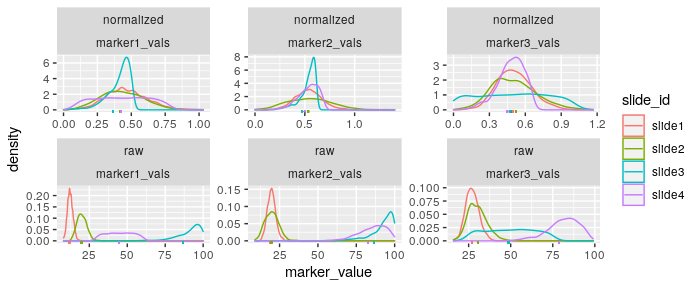plot_mx_discordance(mx_flex_q50)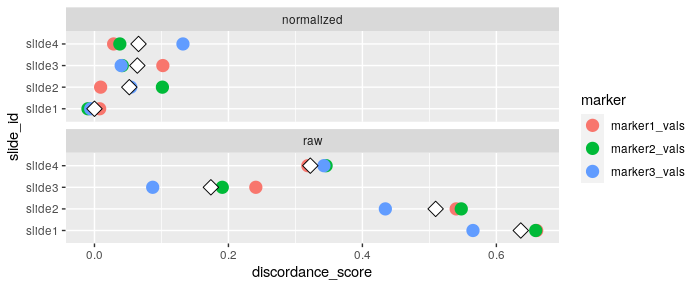Now let’s define the “thresholding” function to return the 10th percentile for our mx_flex_q75 analysis, ensuring that we include a thold_data parameter: q10_threshold = function(thold_data,ptile=0.10){ quantile(thold_data, ptile) } And now let’s run the discordance analysis, with the goal of understanding the change in distance between 10th percentiles in the normalized and unnormalized data. mx_flex_q75 = run_otsu_discordance(mx_flex_q75,table = "both",threshold_override = q10_threshold) summary(mx_flex_q75) #> Call: #> mx_dataset object with 4 slide(s), 3 marker column(s), and 1 metadata column(s) #> #> Normalization: #> Data normalized with transformation=None and method=75th_percentile #> #> Anderson-Darling tests: #> table mean_test_statistic mean_std_test_statistic mean_p_value #> normalized 30.242 20.809 0 #> raw 32.490 22.525 0 #> #> Threshold discordance scores: #> table mean_discordance sd_discordance #> normalized 0.092 0.059 #> raw 0.149 0.098 Although here we are asking a distinct question, e.g. the change in slide-to-slide thresholds for the lower tail of the normalized and unnormalized data, we do see improvements in this metric for the 75th percentile normalization method. plot_mx_density(mx_flex_q75)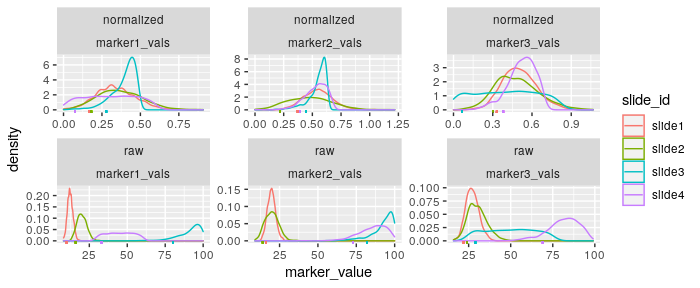plot_mx_discordance(mx_flex_q75)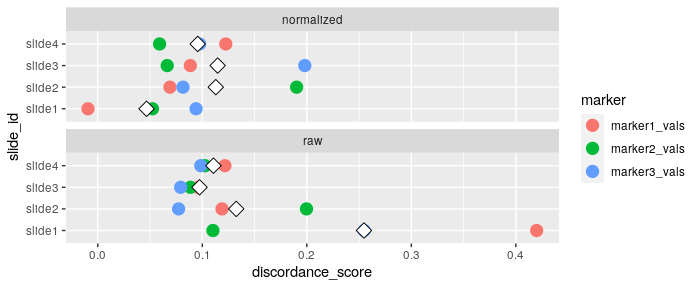### User-defined random effects modeling Taking the mx_flex_q50 object from above, suppose we are interested in understanding the slide-to-slide variation via a random effects model that is not merely the marker intensity values captured by a slide-level random intercept, e.g. marker ~ (1 | slide_id). Given the columns available in our dataset, head(mx_sample,0) #>  slide_id image_id marker1_vals marker2_vals marker3_vals #>  metadata1_vals #> <0 rows> (or 0-length row.names) Let us re-imagine the random effects model as follows, $\text{marker} \sim \text{metadata1_vals} + (1 | \text{image_id})+(1 | \text{slide_id})$ Note that in this simulated scenario these additional covariates are likely unhelpful in terms of creating a robust statistical model, but this provides a good foundation for performing this modeling on real data. Now we can specify the RHS of this model for use in the mxnorm functions like so: new_RHS = "metadata1_vals + (1 | image_id) + (1 | slide_id)" And now using the run_var_proportions(), we can specify the formula_override with this new formula, and set the save_models to TRUE so we can inspect these models later. mx_flex_q50 = run_var_proportions(mx_flex_q50, table="both", formula_override = new_RHS, save_models=TRUE) First let’s look at one of the models we’ve saved, this one for the marker1_vals, which provides some context to what is relevant in the unnormalized models (slide_id in particular), and what is not relevant (e.g., image_id): summary(mx_flex_q50$var_models[])
#> Linear mixed model fit by REML ['lmerMod']
#> Formula: marker1_vals ~ metadata1_vals + (1 | image_id) + (1 | slide_id)
#>    Data: dat
#>
#> REML criterion at convergence: 19482
#>
#> Scaled residuals:
#>     Min      1Q  Median      3Q     Max
#> -6.1732 -0.3660  0.0027  0.5612  2.4344
#>
#> Random effects:
#>  Groups   Name        Variance Std.Dev.
#>  slide_id (Intercept) 1257.7   35.464
#>  image_id (Intercept)    0.0    0.000
#>  Residual               38.3    6.189
#> Number of obs: 3000, groups:  slide_id, 4; image_id, 3
#>
#> Fixed effects:
#>                   Estimate Std. Error t value
#> (Intercept)        42.2934    17.7326   2.385
#>
#> Correlation of Fixed Effects:
#>             (Intr)
#> mtdt1_vlsys -0.007
#> optimizer (nloptwrap) convergence code: 0 (OK)
#> boundary (singular) fit: see ?isSingular

These insights carry through to the mxnorm summaries of the data as well:

summary(mx_flex_q50)
#> Call:
#> mx_dataset object with 4 slide(s), 3 marker column(s), and 1 metadata column(s)
#>
#> Normalization:
#> Data normalized with transformation=None and method=median_divide
#>
#> Anderson-Darling tests:
#>       table mean_test_statistic mean_std_test_statistic mean_p_value
#>  normalized              37.245                  26.157            0
#>         raw              32.490                  22.525            0
#>
#> Threshold discordance scores:
#>       table mean_discordance sd_discordance
#>  normalized            0.045          0.046
#>         raw            0.410          0.191
#>
#> Variance proportions (slide-level):
#>       table  mean    sd
#>  normalized 0.008 0.010
#>         raw 0.940 0.055
plot_mx_proportions(mx_flex_q50)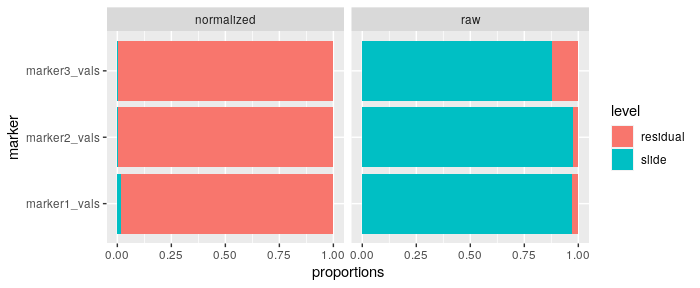Note that since the metadata and IDs are simulated, this more specified random effects model does not necessarily perform better than the initial simple model, but does point to the flexibility of analyzing the results of a normalization method using mxnorm.

## Methodology background

In the mxnorm package, we adapt multiple normalization methods from existing literature and R software (R Core Team 2020) into the multiplexed imaging space. Here we break down the methodological foundations of some of the normalization algorithms used here (ComBat and functional data registration), as well as the mathematical background of some metrics & statistical quantities used in the package to quantify effective normalization techniques.

### ComBat

#### Mathematical background of the ComBat algorithm

We adapted the empirical Bayes framework of the ComBat algorithm (Fortin et al. 2017; Johnson, Li, and Rabinovic 2007) for multiplexed imaging data. Empirical Bayesian methodology can best be described as an alternative to Bayesian analysis, with the analyst assuming priors on the parameters of interest and deriving posterior distributions to collect results, but estimation procedures for the hyper-parameters are informed by the data used in the modeling process. Namely, esteemed statistician George Casella describes the empirical Bayes paradigm as follows:

The empirical Bayesian agrees with the Bayes model but refuses to specify values for [the hyper-parameters]. Instead, he estimates these parameters from the data. (Casella 1985)

Building upon the ComBat algorithm, which itself assumes that batch effects in microarray data can be corrected with a standardization procedure for the mean and variance across batches (Johnson, Li, and Rabinovic 2007), we can parameterize mean and variance of the slide-level batch effects, with the location-scale model as follows (Harris et al. 2022):

$Y_{ic}(u) = \alpha_c + \gamma_{ic} + \delta_{ic} \varepsilon_{ic}(u).$

where we define $$Y_{ic}(u)$$ as the intensity of unit $$u$$ on slide $$i$$ for marker channel $$c$$ and $$\alpha_c$$ as the the grand mean of $$Y_{ic}(u)$$ for channel $$c$$, where $$\gamma_{ic}$$ is the mean batch effect of slide $$i$$ for channel $$c$$ and $$\delta_{ic}^2$$ is the variance batch effect of slide $$i$$ for channel $$c$$. Though in principle, units can be at the pixel or cell level, in our application, $$Y_{ic}(u)$$ is the median cell intensity (or its transformed counterpart) of a selected marker for a given segmented cell on a specific slide in the dataset. We calculate the hyper-parameter estimates using the method of moments and iterate between estimating the hyper-parameters and batch effect parameters until convergence. Upon convergence, we use these batch effects, $$\hat\gamma_{ic}^*$$ and $$\hat\delta_{ic}^*$$, to adjust the data, $Y_{ic}^*(u) = \frac{\hat\sigma_c^2}{\hat\delta_{ic}^*}(Z_{ic}(u) - \hat\gamma_{ic}^*) + \hat\alpha_c.$

Here we define $$\hat\sigma_c = \frac{1}{N}\sum_{ic}(Y_{ic}(u) -\hat\alpha_c - \hat\gamma_{ic})^2$$ from the data and let:

$Z_{ic}(u) = \frac{Y_{ic}(u) -\hat\alpha_c}{\hat\sigma_c^2},$

In short, this model adjusts the Z-normalized intensity data, $$Z_{ic}(u)$$, by the mean and variance batch effects, and re-scales back to the initial scale of the data with the mean and variance of the raw marker intensity values.

#### Existing implementations of ComBat

The original ComBat algorithm is implemented in the Surrogate Variable Analysis (sva) Bioconductor package, which is a popular and well-maintained package “for removing batch effects and other unwanted variation in high-throughput experiment” (Leek et al. 2020). The ComBat function is well-documented and versatile for correcting batch effects using the method introduced originally in microarray data via sva::ComBat(), however, the assumptions made for this function are based largely on the expression matrices produced in microarray studies, not those typical to imaging or multiplexed studies.

Efforts to extend into the neouroimaging space provide a good foundation for adapting the ComBat algorithm to alternate modalities (Fortin et al. 2017), which inspired our extension into the multiplexed domain. Our implementation here is similar to that adapted by Fortin et al in the neuroCombat package, but is focused largely on datasets typical in the multiplexed imaging field.

#### The mxnorm implementation of ComBat

There are a handful of distinctions to discuss regarding the ComBat implementation in mxnorm. As noted above in the Overview, we expect multiplexed imaging data to be marker-dependent and in the “long” format. This means that for some set of multiplexed $$n$$ slides and $$m$$ images, we don’t expect a perfect $$n\times m$$ expression matrix for a given marker channel. We can also take advantage of working with “long” data to leverage tidyverse packages & functions like dplyr for easier/faster calculation of batch effects – this algorithm is detailed in the /R/combat_helpers.R file. Ultimately, we then take the same approach with running the ComBat algorithm – initialize values of our parameters of interest, run the algorithm to calculate batch effects using empirical Bayes, and then standardize the data to correct for slide-to-slide variation.

Adjustable hyper-parameters that are available to pass to the mxnorm::mx_normalize() function for the ComBat algorithm include:

• remove_zeroes: boolean to remove zeroes from ComBat analysis (default=TRUE)
• tol: iterative tolerance of ComBat algorithm (default=0.0001)

### Functional data registration

#### Mathematical background of fda registration

The second main algorithm adapted for this package is based upon methods developed in the functional data analysis (FDA) paradigm, which broadly is a field of statistics that seeks to understand differences between curves as defined by a set of dimensions (Ramsay 2004). A registration algorithm is one that assumes some differences between curves based on some variable are due to noise or variation, and defines criteria to “adjust” the curves and align them to some x- and y-coordinates.

In practice, we implement the fda package here to perform these adjustments to the data (Ramsay, Graves, and Hooker 2021). This approach uses FDA methods to approximate histograms as smooth densities, and uses a registration algorithm to align the densities to their average. The actual algorithm is performed by estimating a monotonic warping function for each density that stretches and compresses the domain such that densities are aligned; these warping functions are then used to transform the values.

In our case (Harris et al. 2022), we let our observed cell intensity values $$Y_{ic}(u)$$ from the multiplexed imaging data have density $$Y_{ic}(u) \sim f(y \mid i,c)$$. Our goal is to remove technical variation related to the slide by estimating a monotonic warping function, $$\phi_{ic}(y)$$, and then use a $$k$$ degree of freedom cubic B-spline basis to approximate the densities of the median cell intensities for each slide and marker, $$f(y \mid i, c) \approx \beta^T g(y)$$ where $$\beta \in \mathbb{R}^{k}$$ is an unknown coefficient vector and $$g(y)$$ is a vector of known basis functions.

We then register the approximated histograms to the average, restricting the warping function to be a 2 degree of freedom linear B-spline basis for some functions $$h_1(y)$$ and $$h_2(y)$$ and for constants $$C_0$$ and $$C_1$$ to be estimated from the data, $\phi_{ic}(x) = C_0 + C_1 \int_0^x \exp \left\{\beta_{1ic}h_1(y) + \beta_{2ic} h_2(y)\right\} dy,$ such that the transformation is monotonic (Ramsay 2004). Unknown parameters $$\beta_{1ic}$$ and $$\beta_{2ic}$$ are estimated to minimize the distance between the average registered curve and the registered densities. We can then use the warping function $$\phi_{ic}(y)$$ to calculate the normalized intensity values: $Y^*_{ic}(u) = \phi_{ic}(Y_{ic}(u) )$

In short, we are defining some warping function to transform the raw cell intensity values for some given marker and slide, into normalized intensity values.

#### Existing implementations of registration

While the fda package is the basis of much functional data analysis in R (and the basis of the analyses performed in mxnorm), there are a handful of other implementations/extensions of this field that are relevant to the mxnorm package both for underlying methods and better understanding of functional data. Extensions of the FDA paradigm in R include the refund package (Goldsmith et al. 2021), which includes methods for regression of functional data and similar applications to imaging data, and the registr package (Wrobel et al. 2021) that focuses on the registration of functional data generated from exponential families. There are also similar extensions of registration algorithms like the mica package (Wrobel 2021), which seeks to apply FDA registration algorithms to the harmonization of multisite neuroimaging data.

#### The mxnorm implementation of fda registration

Again as noted in the Overview, we expect multiplexed imaging data to be marker-dependent and in the “long” format – hence we run the registration algorithm across slides for a given marker. Here we are using the fda package to setup the basis functions, run initial registration, generate the inverse warping functions, and then register the raw data to the mean registered curve to create a normalized intensity value. This process and the extensive hyper-parameters available are detailed in the /R/registration_helpers.R file.

Adjustable hyper-parameters that are available to pass to the mxnorm::mx_normalize() function for the fda registration algorithm include:

• len: the number of equally spaced points at which the density is to be estimated (default=512)
• weighted: boolean to determine if weighted mean to be used via mxnorm::weighted.mean.fd() (default=TRUE)
• offset: offset from zero when calculating weights (default=0.0001)
• fdobj_norder: integer specifying the order of b-splines for the histogram approximation (default=4)
• fdobj_nbasis: integer variable specifying the number of basis functions for the histogram approximation (default=21)
• w_norder: integer specifying the order of b-splines for transformation (default=2)
• w_nbasis: integer variable specifying the number of basis functions for the histogram approximation (default=2)

### Evaluation framework

#### Alignment of marker densities

In a successful normalization algorithm, we expect alignment of the density curves of a given marker’s intensity values – to check this assumption, we use the $$k$$-samples Anderson-Darling test statistic to quantify the likelihood that each slide is drawn from the same population (Scholz and Stephens 1987). Higher values of this test statistic indicate greater evidence that the k-samples are drawn from different distributions, so for a “good” normalization method, we expect smaller values of the AD test statistic.

We’ve implemented this test statistic into the summary.mx_dataset() S3 method we developed, and utilize the bkde() density estimate from the KernSmooth package to quickly compute the density cruves, then run the AD test using the kSamples package (Wand 2021; Scholz and Zhu 2019).

#### Threshold discordance and accuracy

Otsu thresholds are a commonly used thresholding algorithm that defines an optimal threshold in gray-scale images and histograms (Otsu 1979), that we use here to define a new metric of measuring agreement of marker intensity values across slides. This metric, termed the Otsu discordance score, measures the slide-to-slide discordance across all markers and transformation methods, to determine how similar Otsu thresholds are across slides following normalization. Here, a lower value of the threshold discordance score indicates better agreement across slides in the data. For some unit of measurement $$u$$ for marker channel $$c$$ on slide $$i$$, let $$O_{ic}(u,o)$$ indicate which cells have values greater than the Otsu thresold $$o$$. We then define the discordance metric as follows: $\frac{1}{N}\sum_i^N \left(\frac{\sum_y |O_{ic}(u,o_{ic}) - O_c(u,o_c)|}{U_{ic}}\right)$ Where $$U_{ic}$$ is the number of quantified cells present on a particular slide $$i$$ for a given channel $$c$$, $$o_{ic}$$ is the slide and channel-specific Otsu threshold, and $$o_c$$ is the threshold estimated across all slides for a given channel.

We implement this metric in the mxnorm package as an analysis method, e.g. run_otsu_discordance(), which takes in the mx_dataset object and produces an output table in the mx_dataset object called otsu_data which has the following structure:

slide_id | marker | table | slide_threshold | marker_threshold | discordance_score

The mean and SD of the discordance is also produced when summarizing the object using summary.mx_dataset() for a given mx_dataset object if the Otsu discordance analysis has already been run.

To calculate Otsu thresholds in our package, we use the thresholding options from the scikit-image.filters Python module which provide a notable speed increase on Otsu thresholding methods available in R (Walt et al. 2014). Note that thresholding options extend beyond just the Otsu threshold – the discordance score can be overridden to either accept an user-defined thresholding method or one of the univariate thresholds from scikit-image.filters.

#### Proportions of variance

We also include an analysis method to assess a normalization method’s ability to remove slide-related variance, using random effects modeling in the lme4 package (Bates, Maechler, and Bolker 2015). The default analysis fits a model for each marker in the dataset using only a slide-level intercept – this model can include additional covariates when using the metadata_cols parameter or re-define the modeling formula using formula_override.

#### UMAP embedding

The final analysis method included in the package is the UMAP embedding algorithm (McInnes, Healy, and Melville 2018), a dimension reduction commonly used in the biological sciences, here implemented using the uwot R package (Melville 2021). The method is often used to distinguish differences between groups and here can be used to highlight slide effects (clustering of slides) or determine biological separation of groups. These options must be included in the run_reduce_umap() call using the metadata_cols parameter, and then can be visually inspected using the plot_mx_umap() method. Also note that the UMAP algorithm may take up significant computational time for large datasets – we’ve allowed for random downsampling of the data via the downsample_pct parameter to alleviate these concerns.

To further quantify the separation of groups for some given metadata, we implement the Cohen’s kappa metric from the psych package and adjusted Rand index from the fossil package (Revelle 2021; Vavrek 2011). Each of these are executed using summary.mx_dataset() on an mx_dataset object that has already run a UMAP embedding analysis.